# Some Neat Features

Pascal’s Triangle is riddled with intriguing patterns and embedded sequences. Here it is again: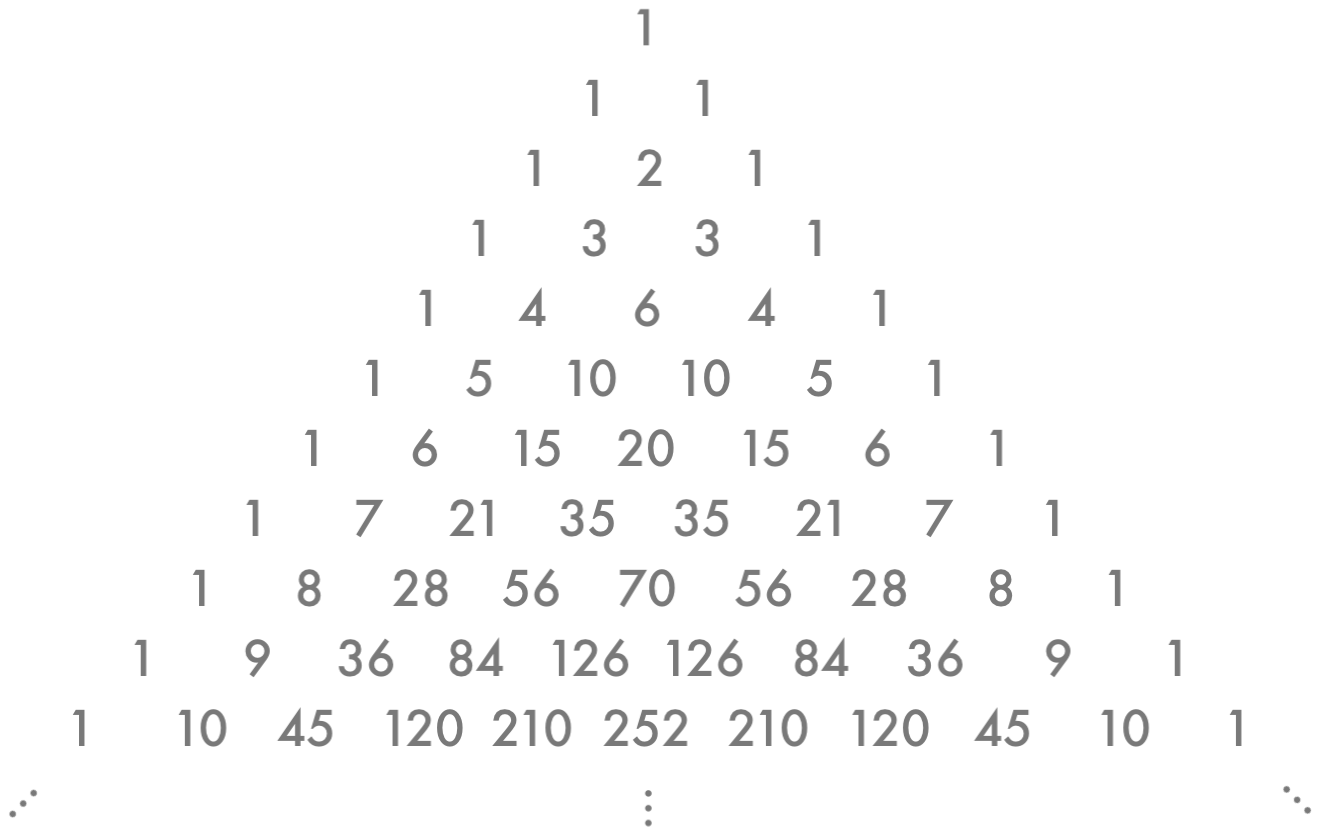For easy reference, we’ll call the lonely 1 at the top Row 0, and the row below that Row 1, then below that Row 2, and so on. Then in each row, the first 1 at the very left will be in Position 0, and the number to its right in Position 1, then to the right Position 2, etc. Then, for instance, the last 10 in “1 5 10 10 5 1” is in Row 5, Position 3. It might feel odd to start counting at 0 this way, but it’ll make sense in a minute.

So – the remarkable properties. Step right up, ladies and gentlemen…

First, notice that Pascal’s triangle is symmetrical. That’s perhaps not too surprising; when we apply the rule that generates the triangle, we’re doing the same thing to both sides of the figure, so there’s no reason for asymmetry.

Then, look at the diagonals. On the far left we have a long string of 1s. (That’s because each one is the sum of the 1 and invisible 0 above it.) Then the second diagonal is made of simply the natural numbers: our counting numbers 1, 2, 3, and so on. Why? Think about it – at every row you’re adding a 1 from the first diagonal. The third diagonal is made of the triangular numbers; I’ll leave ye intrepid readers to puzzle out why on your own.

Next, let’s color Pascal’s Triangle. We’ll put a blue dot on every odd number and a gray dot on every even number.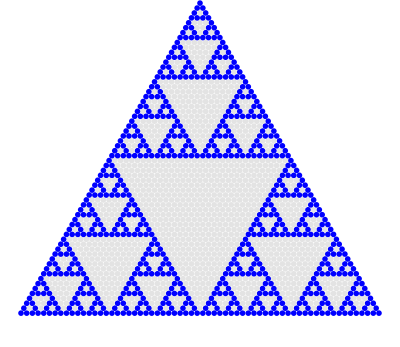Image by Brent Yorgey / CC BY NC 3.0

Hm, looks rather like our friend Sierpinski…. In fact, if you continue this coloring pattern on to infinity and squash it into a finite perimeter, it is the Sierpinski Triangle.

Here’s another nifty thing about Pascal’s Triangle: Add up the numbers in each row and see what you get.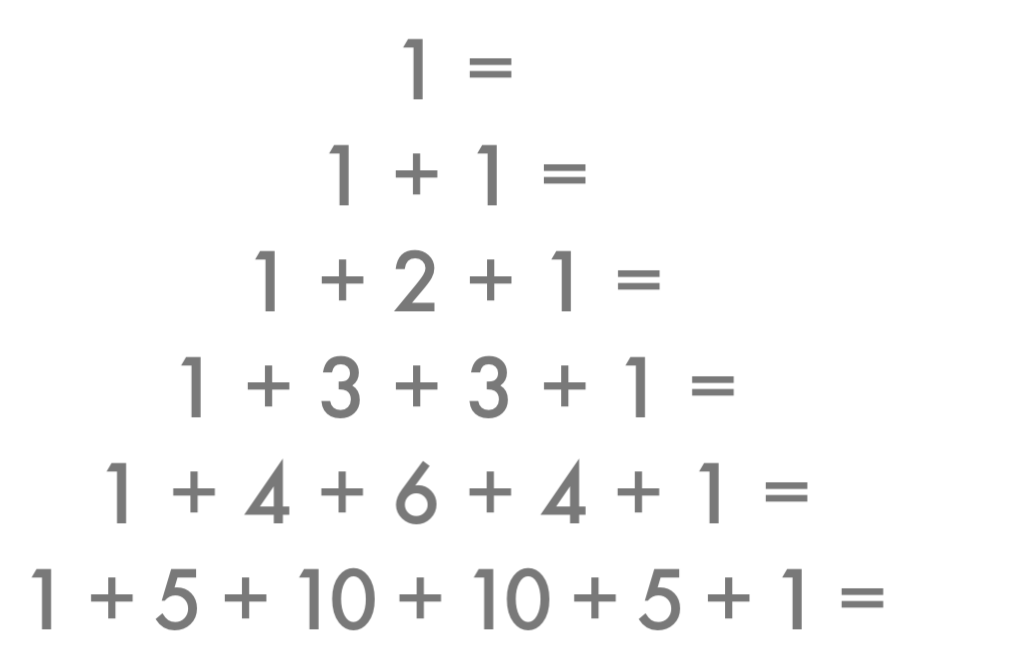Recognize those numbers? They’re the powers of 2. Specifically, the $n$th row of Pascal’s Triangle adds up to $2^n$.

Try this. For every row, insert plus and minus signs between the numbers, starting with a – and alternating from there: –, +, –, +, –, +, –, +, …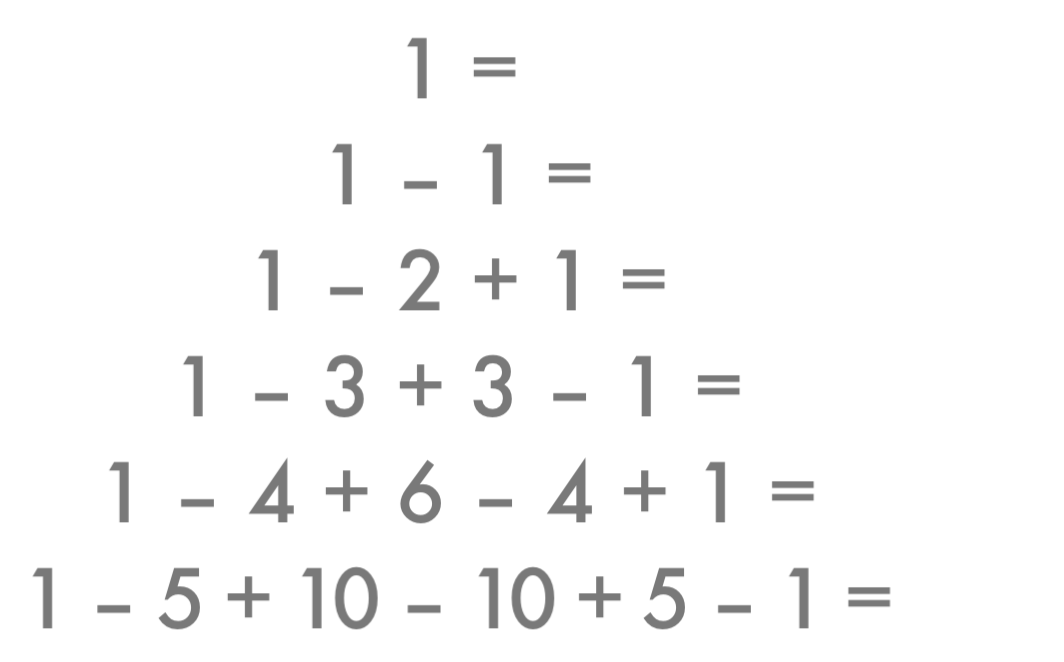Except for the lonely 1 at the top, the rows thus evaluated are equal to 0.

For our next trick, we’ll need to shift Pascal’s Triangle so it all lines up along a left boundary.Now we’ll mark the diagonals and add them up: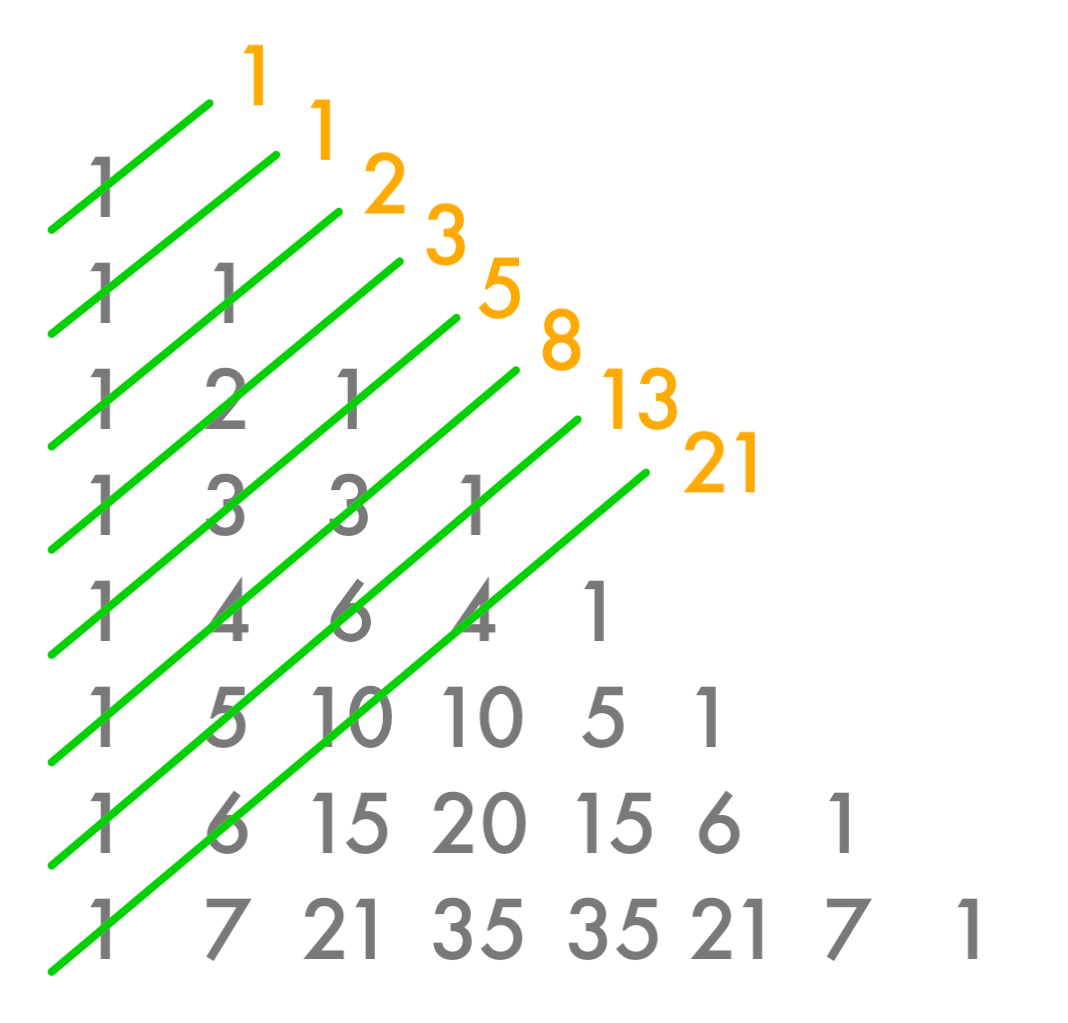And look there, we’ve uncovered our good friends the Fibonacci numbers!

These properties are pretty remarkable all right, but we have yet to explore the most remarkable property of all